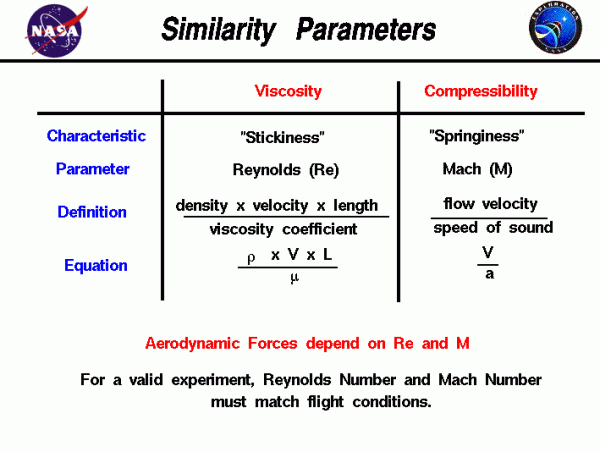# Similarity parameters## Manipulated Variables of Rockets

As a rocket moves through the atmosphere, the gas molecules of the atmosphere near the rocket are disturbed and move around the rocket. Aerodynamic forces are generated between the gas and the rocket. The magnitude of these forces depend on the shape of the rocket, the speed of the rocket, the mass of the gas going by the rocket and on two other important properties of the gas; the viscosity, or stickiness, of the gas and the compressibility, or springiness, of the gas. To properly model these effects, aerodynamicists use similarity parameters, which are ratios of these effects to other forces present in the problem. If two experiments have the same values for the similarity parameters, then the relative importance of the forces are being correctly modeled. Representative values for the properties of the Earth’s atmosphere and the Martian atmosphere are given on other pages, but the actual value of the parameter depends on the state of the gas and on the altitude.

## Disarrays in Launching Rockets

Aerodynamic forces depend in a complex way on the viscosity of the gas. As a rocket moves through a gas, the gas molecules stick to the surface. This creates a layer of air near the surface, called a boundary layer, which, in effect, changes the shape of the rocket. The flow of gas reacts to the edge of the boundary layer as if it was the physical surface of the rocket. To make things more confusing, the boundary layer may separate from the body and create an effective shape much different from the physical shape. And to make it even more confusing, the flow conditions in and near the boundary layer are often unsteady (changing in time). The boundary layer is very important in determining the drag of an object. To determine and predict these conditions, aerodynamicists rely on wind tunnel testing and very sophisticated computer analysis.

## The Reynolds Number

The important similarity parameter for viscosity is the Reynolds number. The Reynolds number expresses the ratio of inertial (resistant to change or motion) forces to viscous (heavy and gluey) forces. From a detailed analysis of the momentum conservation equation, the inertial forces are characterized by the product of the density r times the velocity V times the gradient of the velocity dV/dx. The viscous forces are characterized by the viscosity coefficient mu times the second gradient of the velocity d^2V/dx^2. The Reynolds number Re then becomes:

### Re = (r * V * L) / mu

where L is some characteristic length of the problem. If the Reynolds number of the experiment and flight are close, then we properly model the effects of the viscous forces relative to the inertial forces. If they are very different, we do not correctly model the physics of the real problem and predict incorrect levels of the aerodynamic forces.

## Further Complexities in the Launch

Aerodynamic forces also depend in a complex way on the compressibility of the gas. As a rocket moves through the gas, the gas molecules move around the rocket. If the rocket passes at a low speed (typically less than 200 mph) the density of the fluid remains constant. But for high speeds, some of the energy of the rocket goes into compressing the fluid and changing the density, which alters the amount of resulting force on the object. This effect becomes more important as speed increases. Near and beyond the speed of sound (about 330 m/s or 700 mph on earth), shock waves are produced that affect the drag of the rocket. Again, aerodynamicists rely on wind tunnel testing and sophisticated computer analysis to predict these conditions.

## Match Number

The important similarity parameter for compressibility is the Mach number – M, the ratio of the velocity of the rocket to the speed of sound a.

### M = V / a

So it is completely incorrect to measure a drag coefficient at some low speed (say 200 mph) and apply that drag coefficient at twice the speed of sound (approximately 1400 mph, Mach = 2.0). The compressibility of the air alters the important physics between these two cases.

The effects of compressibility and viscosity on drag are contained in the drag coefficient. For propulsion systems, compressibility affects the amount of mass that can pass through an engine and the amount of thrust generated by a rocket nozzle.

Provide feedback